# Rbse Solutions Class 6 Maths Chapter 14 – Practical Geometry

Exercise 14.1 page no: 276

1. Draw a circle of radius 3.2 cm.

Solutions:

The required circle may be drawn as follows:

Step 1: For the required radius 3.2 cm, first open the compasses.

Step 2: For the centre of a circle, mark a point ‘O’.

Step 3: Place a pointer of compasses on ‘O’.

Step 4: Now, turn the compasses slowly to draw the required circle.

2. With the same centre O, draw two circles of radii 4 cm and 2.5 cm.

Solutions:

The required circle may be drawn as follows:

Step 1: For the required radius 4 cm, first open the compasses

Step 2: For the centre of a circle, mark a point ‘O’

Step 3: Place a pointer of compasses on ‘O’.

Step 4: Turn the compasses slowly to draw the circle.

Step 5: Next, open the compasses for 2.5 cm.

Step 6: Again place a pointer of compasses on ‘O’ and turn the compasses slowly to draw the circle.

3. Draw a circle and any two of its diameters. If you join the ends of these diameters, what is the figure obtained? What figure is obtained if the diameters are perpendicular to each other? How do you check your answer?

Solutions:

We may draw a circle having its centre ‘O’, also of any convenient radius. Let AB and CD be the two diameters of this circle. A quadrilateral is formed when we join the ends of these diameters.

We know that the diameter of a circle are equal in length, hence quadrilateral formed will be having its diagonals of equal length.

Also, OA = OB = OC = OD = radius r and if a quadrilateral has its diagonals of same length bisecting each other. It will be a rectangle.

Let DE and FG be the diameters of the circle such that both are perpendicular to each other. Now we can find that a quadrilateral is formed by joining the ends of these diameters.

We may find that OD = OE = OF = OG = radius r.

In this quadrilateral DFEG, diagonals are equal and perpendicular to each other. Also they are bisecting each other, hence it will be a square.

To check our answers we may measure the length of sides of quadrilateral formed.

4. Draw any circle and mark points A, B and C such that

(a) A is on the circle.

(b) B is in the interior of the circle.

(c) C is in the exterior of the circle.

Solutions:

We may draw a circle and three required points A, B, C as follows:

5. Let A, B be the centres of two circles of equal radii; draw them so that each one of them passes through the centre of the other. Let them intersect at C and D. Examine whether  and  are at right angles.

Solutions:

Let us draw two circles having with same radius which are passing through the centre of the other circle.

Here points A and B are the centres of these circles and these circles are intersecting each other at points C and D, respectively.

BC = BD [radius of circle centered at B]

Since, radius of both the circles are equal.

Therefore AD = AC = BC = BD

Hence quadrilateral ADBC is a rhombus and in rhombus diagonals bisect each other at 900. Hence  and  are at right angles.

Exercise 14.2 Page no: 278

1. Draw a line segment of length 7.3 cm using a ruler.

Solutions:

Using a ruler, we can draw a line segment of length 7.3 cm as follows

Step 1: Mark a point A on the sheet

Step 2: Place 0 mark of ruler at point A

Step 3: At 7.3 cm on ruler, mark a point B on the sheet

Step 4: Now join A and B

Here  is the required line segment.

2. Construct a line segment of length 5.6 cm using ruler and compasses.

Solutions:

By using a ruler and compasses, we may draw a line segment of length 5.6 cm as follows

Step 1: Draw a line l and mark a point A on this line l.

Step 2: On the zero mark of the ruler, place the compasses. Now extend the compasses to place the pencil up to the 5.6 cm and mark.

Step 3: Place the pointer of compasses on point A and draw an arc to cut l at B. Now,
is the line segment of 5.6 cm length.

3. Construct  of length 7.8 cm. From this, cut off  of length 4.7 cm. Measure .

Solutions:

Step 1: Draw a line l and mark a point A on it.

Step 2: By adjusting the compasses up to 7.8 cm, while putting the pointer of compasses on point A draw an arc to cut l on B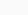is the line segment of 7.8 cm

Step 3: By adjusting the compasses up to 4.7 cm, draw an arc to cut l on C, while putting the pointer of compasses on point A.is the line segment of of 4.7 cm

Step 4: Now, place the ruler in such a way that 0 mark of the ruler will match with the point C.

Now read the position of point B. It will be 3.1 cm.

is 3.1 cm

4. Given  of length 3.9 cm, construct  such that the length of  is twice that of . Verify by measurement.

Solutions:

We can draw a line segment  such that the length of  is twice that of  as follows

(1) Draw a line l and mark a point P on it. Let AB be the given line segment of 3.9 cm.

(2) By adjusting the compasses up to the length of AB, place the pointer of compasses at point P and draw an arc to cut the line at X.

(3) Again place the pointer on point X, draw an arc to cut line l at point Q.

is the required line segment. Using the ruler we may measure the length of
which comes to 7.8 cm.

5. Given  of length 7.3 cm and  of length 3.4 cm, construct a line segment such that the length of  is equal to the difference between the lengths of  and  . Verify by measurement.

Solutions:

(1) Given  = 7.3 cm and  = 3.4 cm

(2) Adjust the compasses up to the length of CD and put the pointer of compasses at point A, draw an arc to cut AB at point P.

(3) Adjust the compasses up to the length of PB. Draw a line l and mark a point X on it.

(4) By placing the pointer of compasses at point X, draw an arc to cut the line at Y.

is the required line segment

Now, difference between the lengths of  and  = 7.3 – 3.4 = 3.9 cm

Using the ruler we may measure the length of  which comes to 3.9 cm.

Exercise 14.3 page no: 279

1. Draw any line segment. Without measuring, construct a copy of .

Solutions:

(1) Let the given line segment be

(2) Adjust the compasses up to the length of

(3) Draw any line l and mark a point A on it

(4) Place the pointer on point A, without changing the setting of compasses, draw an arc to cut the line segment at B point.

is the required line segment

2. Given some line segment, whose length you do not know, construct  such that the length of  is twice that of .

Solutions:

Following steps are followed to construct a line segment  such that the length of  is twice that of

(1) Let the given line segment be

(2) Adjust the compasses up to the length of

(3) Draw a line l and mark a point P on it

(4) Place the pointer on P, draw an arc to cut the line segment at point X, without changing the setting of compasses

(5) Again with same radius as before, by placing the pointer on point X, draw an arc to cut the line l at point Q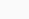is the required line segment.

Exercise 14.4 Page no: 284

1. Draw any line segment . Mark any point M on it. Through M, draw a perpendicular to . (use ruler and compasses)

Solutions:

(1) Draw a line segment  and mark a point M on it.

(2) Taking M as centre and a convenient radius, construct an arc intersecting the line segment
at points C and D, respectively.

(3) By taking centres as C and D and radius greater than CM, construct two arcs such that they intersect each other at point E.

(4) Join EM. Now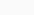is perpendicular to

2. Draw any line segment . Take any point R not on it. Through R, draw a perpendicular to . (use ruler and set-square)

Solutions:

(1) Draw a given line segment  and mark a point R outside the line segment

(2) Place a set square on  such that one of its right angles arm aligns along

(3) Now, place the ruler along the edge opposite to right angle of set square.

(4) Hold the ruler fixed. Slide the set square along the ruler such that the point R touches the other arm of set square.

(5) Draw a line along this edge of set square which passes through point R. Now, it is the required line perpendicular to

3. Draw a line l and a point X on it. Through X, draw a line segment perpendicular to l.

Now draw a perpendicular to XY at Y. (use ruler and compasses)

Solutions:

(1) Draw a line l and mark a point X on it.

(2) By taking X as centre and with a convenient radius, draw an arc intersecting the line l at points A and B, respectively.

(3) With A and B as centres and a radius more than AX, construct two arcs such that they intersect each other at point Y.

(4) Join XY. Here  is perpendicular to lSimilarly, by taking C and D as centres and radius more than CY, construct two arcs intersecting at point Z. Join ZY. The lineis perpendicular to  at Y

Exercise 14.5 page no: 286

1. Drawof length 7.3 cm and find its axis of symmetry.

Solutions:

Following steps are followed to constructof length 7.3 cm and to find its axis of symmetry

(1) Draw a line segmentof 7.3 cm

(2) Take A as centre and draw a circle by using compasses. The radius of circle should be more than half the length of.

(3) Now, take B as centre and draw another circle using compasses with the same radius as before. Let it cut the previous circle at points C and D

(4) Join CD. Now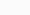is the axis of symmetry

2. Draw a line segment of length 9.5 cm and construct its perpendicular bisector.

Solutions:

Following steps are observed to construct a line segment of length 9.5 cm and to construct its perpendicular bisector

(1) Draw a line segmentof 9.5 cm

(2) Take point P as centre and draw a circle by using compasses. The radius of circle should be more than half the length of(3) Taking the centre at point Q, again draw another circle using compasses with the same radius as before. Let it cut the previous circle at R and S respectively.

(4) Join RS. Now,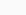is the axis of symmetry i.e the perpendicular bisector of the line3. Draw the perpendicular bisector of whose length is 10.3 cm.

(a) Take any point P on the bisector drawn. Examine whether PX = PY.

(b) If M is the mid point of , what can you say about the lengths MX and XY?

Solutions:

(1) Draw a line segment  of 10.3 cm

(2) Take point X as centre and draw a circle by using compasses. The radius of circle should be more than half the length of

(3) Now taking Y as centre, draw another circle using compasses with the same radius as before. Let it cut at previous circle at points A and B

(4) Join AB. Hereis the axis of symmetry

(a) Take any point P on. We may observe that the measure of lengths of PX and PY are samebeing the axis of symmetry, any point lying onwill be at same distance from the both ends of

(b) M is the midpoint of . Perpendicular bisectorwill be passing through point M. Hence length of  is double of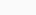or 2MX = XY.

4. Draw a line segment of length 12.8 cm. Using compasses, divide it into four equal parts. Verify by actual measurement.

Solutions:

(1) Draw a line segment  of 12.8 cm

(2) By taking point X as centre and radius more than half of XY, draw a circle.

(3) Again with same radius and centre as Y, draw two arcs to cut the circle at points A and B. Join AB which intersects  at point M

(4) By taking X and Y as centres, draw two circles with radius more than half of(5) Taking M as centre and with same radius, draw two arcs to intersect these circles at P, Q and R, S

(6) Join PQ, and RS. These are intersecting  at points T and U.

(7) The 4 equal parts of  are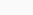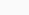BY measuring these line segments with the help of a ruler, we may observe that each is of 3.2 cm

5. With  of length 6.1 cm as diameter, draw a circle.

Solutions:

(1) Draw a line segment  of 6.1 cm

(2) Take point P as centre and radius more than half of , draw a circle

(3) Again with same radius and Q as centre, draw two arcs intersecting the circle at points R and S

(4) Join RS which intersects  at T.

(5) Taking the centre as T and radius TP, draw a circle which passes through Q. Now, this is the required circle.

6. Draw a circle with centre C and radius 3.4 cm. Draw any chord . Construct the perpendicular bisector of  and examine if it passes through C.

Solutions:

(1) Mark any point C on the sheet

(2) Adjust the compasses up to 3.4 cm and by putting the pointer of compasses at point C, turn compasses slowly to draw the circle. This is the required circle of 3.4 cm radius.

(3) Mark any chord  in the circle

(4) Now, taking A and B as centres, draw arcs on both sides of . Let these intersect each other at points D and E.

(5) Join DE. Now DE is the perpendicular bisector of AB.

Ifis extended, it will pass through point C.

7. Repeat Question 6, if  happens to be a diameter.

Solutions:

(1) Mark any point C on the sheet.

(2) Adjust the compasses up to 3.4 cm and by putting the pointer of compasses at point C, Turn the compasses slowly to draw the circle. This is the required circle of 3.4 cm

(3) Now mark any diameter  in the circle.

(4) Now taking A and B as centres, draw arcs on both sides of  with radius more than . Let these intersect each other at points D and E.

(5) Join DE, which is perpendicular bisector of AB.

Now, we may observe thatis passing through the centre C of the circle.

8. Draw a circle of radius 4 cm. Draw any two of its chords. Construct the perpendicular bisectors of these chords. Where do they meet?

Solutions:

(1) Mark any point O on the sheet. Now adjust the compasses up to 4 cm and by placing the pointer of compasses at point O, turn the compasses slowly to draw the circle. This is the required circle of 4 cm radius

(2) Take any two chords  andin the circle

(3) By taking A and B as centres and radius more than half of , draw arcs on both sides of AB. The arcs are intersecting each other at point E and F. Join EF which is perpendicular bisector of AB.

(4) Again take C and D as centres and radius more than half of, draw arcs on both sides of CD such that they are intersecting each other at points G, H. Join GH which is perpendicular bisector of CD

We may observe that when EF and GH are extended they meet at the point O, which is the centre of circle

9. Draw any angle with vertex O. Take a point A on one of its arms and B on another such that OA = OB. Draw the perpendicular bisectors of  and . Let them meet at P. Is PA = PB?

Solutions:

(1) Draw any angle with vertex as O.

(2) By taking O as centre and with convenient radius, draw arcs on both rays of this angle. Let these points are A and B

(3) Now take O and A as centres and with radius more than half of OA, draw arcs on both sides of OA. Let these intersects at points C and D respectively. Join CD

(4) Similarly we may findwhich is perpendicular bisector of . These perpendicular bisectorsandintersects each other at point P. Now measure PA and PB. They are equal in length.

Exercise 14.6 page no: 291

1. Draw ∠POQ of measure 75° and find its line of symmetry.

Solutions:

Following steps are followed to construct an angle of 750 and its line of symmetry

(i) Draw a line l and mark two points O and Q on it. Draw an arc of convenient radius, while taking centre as O. Let this intersect line l at R

(ii) Taking R as centre and with same radius as before, draw an arc such that it is intersecting the previously drawn arc at S

(iii) By taking same radius as before and S as centre, draw an arc intersecting the arc at point T as shown in figure

(iv) Take S and T as centre, draw an arc of same radius such that they intersect each other at U

(v) Join OU. Let it intersect the arc at V. Now, take S and V as centres draw arcs with radius more than 1 / 2 SV. Let these intersect each other at P. Join OP. Now OP is the ray making 750 with the line l.

(vi) Let this ray intersect our major arc at point W. By taking R and W as centres, draw arcs with radius more than 1 / 2 RW in the interior angle of 750. Let these intersect each other at point X. Join OX

OX is the line of symmetry for the ∠POQ = 750

2. Draw an angle of measure 147° and construct its bisector.

Solutions:

Following steps are followed to construct an angle of measure 1470 and its bisector

(i) Draw a line l and mark point O on it. Place the centre of protractor at point O and the zero edge along line l

(ii) Mark a point A at an angle of measure 1470. Join OA. Now OA is the required ray making 1470 with line l

(iii) By taking point O as centre, draw an arc of convenient radius. Let this intersect both rays of angle 1470 at points A and B.

(iv) By taking A and B as centres draw arcs of radius more than 1 / 2 AB in the interior angle of 1470. Let these intersect each other at point C. Join OC.

OC is the required bisector of 1470 angle

3. Draw a right angle and construct its bisector.

Solutions:

Following steps are followed to construct a right angle and its bisector.

(i) Draw a line l and mark a point P on it. Draw an arc of convenient radius by taking point P as centre. Let this intersect line l at R

(ii) Draw an arc by taking R as centre and with the same radius as before such that it is intersecting the previously drawn arc at S

(iii) Take S as centre and with the same radius as before, draw an arc intersecting the arc at T as shown in figure

(iv) By taking S and T as centres draw arcs of same radius such that they are intersecting each other at U.

(v) Join PU. PU is the required ray making a right angle with the line l. Let this intersect major arc at point V.

(vi) Now take R and V as centres, draw arcs with radius more than 1 / 2 RV to intersect each other at point W. Join PW.

PW is the required bisector of this right angle.

4. Draw an angle of measure 153° and divide it into four equal parts.

Solutions:

Following steps are followed to construct an angle of measure 1530 and its bisector

(i) Draw a line l and mark a point O on it. Place the centre of protractor at point O and the zero edge along line l

(ii) Mark a point A at the measure of angle 1530. Join OA. Now OA is the required ray making 1530 with line l

(iii) Draw an arc of convenient radius by taking point O as centre. Let this intersects both rays of angle 1530 at points A and B.

(iv) Take A and B as centres and draw arcs of radius more than 1 / 2 AB in the interior of angle of 1530. Let these intersect each other at C. Join OC

(v) Let OC intersect major arc at point D. Draw arcs of radius more than 1 / 2 AD with A and D as centres and also D and B as centres. Let these are intersecting each other at points E and F respectively. Now join OE and OF

OF, OC, OE are the rays dividing 1530 angle into four equal parts.

5. Construct with ruler and compasses, angles of following measures:

(a) 60°

(b) 30°

(c) 90°

(d) 120°

(e) 45°

(f) 135°

Solutions:

(a) 600

Following steps are followed to construct an angle of 600

(i) Draw a line l and mark a point P on it. Take P as centre and with convenient radius, draw an arc of a circle such that it intersects the line l at Q.

(ii) Take Q as centre and with the same radius as before, draw an arc intersecting the previously drawn arc at point R.

(iii) Join PR. PR is the required ray making 600 with the line l.(b) 300

Following steps are followed to construct an angle of 300

(i) Draw a line l and mark a point P on it. By taking P as centre and with convenient radius, draw an arc of a circle such that it is intersecting the line l at Q.

(ii) Take Q as centre and with the same radius as before, draw an arc intersecting the previously drawn arc at point R.

(iii) By taking Q and R as centres and with radius more than 1 / 2 RQ draw arcs such that they are intersecting each other at S. Join PS which is the required ray making 300 with the line l.

(c) 900

Following steps are followed to construct an angle of measure 900

(i) Draw a line l and mark a point P on it. Take P as centre and with convenient radius, draw an arc of a circle such that it is intersecting the line l at Q.

(ii) Take Q as centre and with the same radius as before, draw an arc intersecting the previously drawn arc at R

(iii) By taking R as centre and with the same radius as before, draw an arc intersecting the arc at S as shown in figure

(iv) Now take R and S as centre, draw arc of same radius to intersect each other at T.

(v) Join PT, which is the required ray making 900 with the line l.

(d) 1200

Following steps are followed to construct an angle of measure 1200

(i) Draw a line l and mark a point P on it. Taking P as centre and with convenient radius, draw an arc of circle such that it is intersecting the line l at Q.

(ii) By taking Q as centre and with the same radius as before, draw an arc intersecting the previously drawn arc at R.

(iii) Take R as centre and with the same radius as before, draw an arc such that it is intersecting the arc at S as shown in figure.

(iv) Join PS, which is the required ray making 1200 with the line l

(e) 450

Following steps are followed to construct an angle of measure 450

(i) Draw a line l and mark a point P on it. Take P as centre and with convenient radius, draw an arc of a circle such that it is intersecting the line l at Q.

(ii) Take Q as centre and with the same radius as before, draw an arc intersecting the previously drawn arc at R

(iii) By taking R as centre and with the same radius as before, draw an arc such that it is intersecting the arc at S as shown in figure.

(iv) Take R and S as centres and draw arcs of same radius such that they are intersecting each other at T

(v) Join PT. Let this intersect the major arc at point U.

(vi) Now take Q and U as centres and draw arcs with radius more than 1 / 2 QU to intersect each other at point V. Join PV.

PV is the required ray making 450 with the line l

(f) 1350

Following steps are followed to construct an angle of measure 1350

(i) Draw a line l and mark a point P on it. Taking P as centre and with convenient radius, draw a semicircle which intersects the line l at Q and R respectively.

(ii) By taking R as centre and with the same radius as before, draw an arc intersecting the previously drawn arc at S

(iii) Taking S as centre and with the same radius as before, draw an arc such that it is intersecting the arc at T as shown in figure

(iv) Take S and T as centres, draw arcs of same radius to intersect each other at U.

(v) Join PU. Let this intersect the arc at V. Now take Q and V as centres and with radius more than 1 / 2 QV, draw arcs to intersects each other at W.

(vi) Join PW which is the required ray making 1350 with the line l

6. Draw an angle of measure 45° and bisect it.

Solutions:

Following steps are followed to construct an angle of measure 450 and its bisector.

(i) Using the protractor ∠POQ of 450 measure may be formed on a line l

(ii) Draw an arc of convenient radius with centre as O. Let this intersects both rays of angle 450 at points A and B

(iii) Take A and B as centres, draw arcs of radius more than 1 / 2 AB in the interior of angle of 450. Let these intersect each other at C. Join OC

OC is the required bisector of 450 angle

7. Draw an angle of measure 135° and bisect it.

Solutions:

Following steps are followed to construct an angle of measure 1350 and its bisector.

(i) By using a protractor ∠POQ of 1350 measure may be formed on a line l

(ii) Draw an arc of convenient radius by taking O as centre. Let this intersect both rays of angle 1350 at points A and B respectively.

(iii) Take A and B as centres, draw arcs of radius more than 1 / 2 AB in the interior of angle of 1350. Let these intersect each other at C. Join OC.

OC is the required bisector of 1350 angle

8. Draw an angle of 700. Make a copy of it using only a straight edge and compasses.

Solutions:

Following steps are followed to construct an angle of measure 700 and its copy.

(i) Draw a line l and mark a point O on it. Now place the centre of protractor at point O and the zero edge along line l.

(ii) Mark a point A at an angle of measure 700. Join OA. Now OA is the ray making 700 with line l. With point O as centre, draw an arc of convenient radius in the interior of 700 angle. Let this intersects both rays of angle 700 at points B and C respectively

(iii) Draw a line m and mark a point P on it. Again draw an arc with same radius as before and P as centre. Let it cut the line m at point D

(iv) Adjust the compasses up to the length of BC. With this radius draw an arc taking D as centre which intersects the previously drawn arc at point E.

(v) Join PE. Here PE is the required ray which makes same angle of measure 700 with the line m

9. Draw an angle of 400. Copy its supplementary angle.

Solutions:

Following steps are followed to construct an angle of measure 450 and a copy of its supplementary angle

(i) Draw a line segmentand mark a point O on it. Place the centre of protractor at point O and the zero edge along line segment.

(ii) Mark a point A at an angle of measure 400. Join OA. Here OA is the required ray making 400 with. ∠POA is the supplementary angle of 400

(iii) With point O as centre, draw an arc of convenient radius in the interior of ∠POA. Let this intersects both rays of ∠POA at points B and C respectively.

(iv) Draw a line m and mark a point S on it. Again draw an arc by taking S as centre with the same radius as used before. Let it cut the line m at point T.

(v) Now adjust the compasses up to the length of BC. Taking T as centre draw an arc with this radius which will intersect the previously drawn arc at point R.

(vi) Join RS. Here RS is the required ray which makes same angle with the line m, as the supplementary of 400 [i.e 1400]

Patio umbrellas sold at Costco recalled after reports of fires गर्मी में लू लगने से बचाव करेंगे यह खाद्य पदार्थ, आज ही खाना करें शुरू ट्रेन के बीच में ही AC कोच क्यों लगाए जाते हैं? Rbse books for class 1 to 12 hindi medium 2021-22